Next: Shape polarization effect (time-even) Up: Core-polarization effects Previous: Core-polarization effects

## Mass polarization effect (time-even)

This effect can be understood as the self-consistent rearrangement of all nucleons, which is induced by an added or subtracted odd particle, while the shape is constrained to the spherical point and the time-odd mean fields are neglected. One has to keep in mind, that none of the s.p. orbitals of a spherical multiplet is spherically symmetric, and therefore, when a particle in such a state is added to a closed core, the resulting state of an odd nucleus cannot be spherically symmetric, and thus cannot be self-consistent. A self-consistent solution can only be realized within the so-called filling approximation, whereupon one assumes the occupation probabilities of all orbitals belonging to the spherical multiplet of angular momentum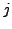and degeneracy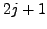, to be equal to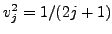.

The mass polarization effect is exactly zero when evaluated within the first-order approximation. Indeed, as expressed by the Koopmans theorem , the linear term in variation of the total energy with respect to adding or subtracting a particle is exactly equal to the bare single-particle energy of the core. However, the obtained results do not agree with Koopmans theorem. This is illustrated in Fig. 1, where we compare bare and polarized s.p. energies of the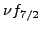and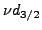orbitals in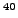Ca. One can see that for both orbitals there is a quite large and positive mass polarization effect.

The reason for this disagreement lies in the fact that, because of the center-of-mass correction, the standard EDF calculations are not really variational with respect to adding or subtracting a particle. Indeed, in these calculations, the total energy of an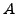-particle system is corrected by the center-of-mass correction [45,46],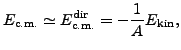(24)

where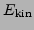is the average kinetic energy of the system. The factor of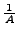is not varied when constructing the standard mean-field Hamiltonian, and therefore, the Koopmans theorem is violated. Had this variation been included, it would have shifted the mean-field potential and thus all bare s.p. energies in given nucleus by a constant,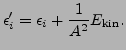(25)

InCa, this shift equals to 0.40MeV and almost exactly corresponds to the entire mass polarization effect shown in Fig. 1. The remaining mass polarization effect can be attributed to higher-order polarization effects (beyond linear) and to the non-self-consistency of the filling approximation.

A few remarks about the shift in Eq. (25) are here in order. First, the effect is independent on whether the two-body or one-body center-of-mass correction  is used, and on whether this is done before or after variation. Only the detailed value of the shift may depend on a particular implementation of the center-of-mass correction. Second, the shift induces an awkward result of the mean-field potential going asymptotically to a positive constant and not to zero. Although this may seem to be a trivial artifact, which does not influence the s.p. wave functions and observables, it shows that the standard center-of-mass correction should be regarded as an ill-defined theoretical construct. This fact shows up as an acute problem in fission calculations . Third, whenever the bare s.p. energies are compared to empirical data, this shift must by taken into account. Alternatively, as advocated in the present study, one should compare directly the calculated and measured mass differences. Finally, one should note that the shift is irrelevant when differences of the s.p. energies are considered, such as the SO splittings discussed in Sec. 4.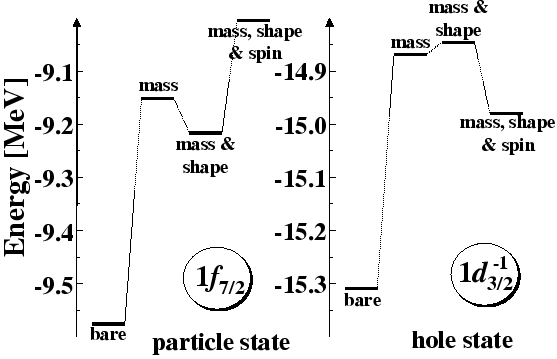Next: Shape polarization effect (time-even) Up: Core-polarization effects Previous: Core-polarization effects
Jacek Dobaczewski 2008-05-18